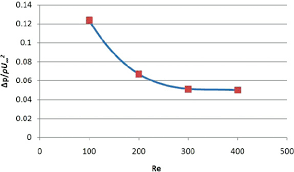## How to Solve and Calculate for a Dimensionless Pressure Drop, Oil Rate, Oil FVF, Oil Viscosity in Well Testing | The Calculator EncyclopediaThe image above represents dimensionless pressure drop.

To compute for the dimensionless pressure drop, five essential parameters are needed and these parameters are Permeability-Thickness Product (kh), Pressure Drop (ΔP), Oil Rate (Qo), Oil Viscosity (μo) and Oil FVF (Bo).

The formula for calculating dimensionless pressure drop:

PD = khΔP / 141.2QoBoμo

Where;

PD = Dimensionless Pressure Drop
kH = Permeability-Thickness Product
ΔP = Pressure Drop
Qo = Oil Rate
μo = Oil Viscosity
Bo = Oil FVF

Let’s solve an example;
Find the dimensionless pressure drop with a permeability-thickness product of 70, a pressure drop of 30, an oil rate of 49, an oil viscosity of 19 and oil FVF of 31.

This implies that;

kh = Permeability-Thickness Product = 20
ΔP = Pressure Drop = 30
Qo = Oil Rate = 49
μo = Oil Viscosity = 19
Bo = Oil FVF = 31

PD = khΔP / 141.2QoBoμo
PD = 20 x 30/141.2 x 49 x 31 x 19
PD = 20 x 30/4075173.19
PD = 600/4075173.19
PD = 0.000147

Therefore, the dimensionless pressure drop is 0.000147 psi.

Calculating the Permeability-Thickness Product when Dimensionless Pressure Drop, Pressure Drop, Oil Rate, Oil Viscosity and Oil FVF.

kh = PD x 141.2QoBoμo / ΔP

Where;

kh = Permeability-Thickness Product
PD = Dimensionless Pressure Drop
ΔP = Pressure Drop
Qo = Oil Rate
μo = Oil Viscosity
Bo = Oil FVF

Let’s solve an example;
Find the permeability-thickness product with a dimensionless pressure drop of 28, a pressure drop of 16, an oil rate of 21, an oil viscosity of 18 and oil FVF of 24 .

This implies that;

PD = Dimensionless Pressure Drop = 28
ΔP = Pressure Drop =16
Qo = Oil Rate = 21
μo = Oil Viscosity = 18
Bo = Oil FVF = 24

kh = PD x 141.2QoBoμo / ΔP
kh = 28 x 141.2 x 21 x 24 x 18 / 16
kh = 35867059.8 / 16
kh = 2241691.2

Therefore, the permeability-thickness product is 2241691.2.

Calculating the Pressure Drop when Dimensionless Pressure Drop, Permeability-Thickness Product, Oil Rate, Oil Viscosity and Oil FVF.

ΔP = PD x 141.2QoBoμo / kh

Where;

ΔP = Pressure Drop
PD = Dimensionless Pressure Drop
kh = Permeability-Thickness Product
Qo = Oil Rate
μo = Oil Viscosity
Bo = Oil FVF

Let’s solve an example;
Find the pressure drop with a dimensionless pressure drop of 38, a permeability-thickness product of 24, an oil rate of 11, an oil viscosity of 8 and oil FVF of 12.

This implies that;

PD = Dimensionless Pressure Drop = 38
kh = Permeability-Thickness Product = 24
Qo = Oil Rate = 11
μo = Oil Viscosity = 8
Bo = Oil FVF = 12

ΔP = PD x 141.2QoBoμo / kh
ΔP = 38 x 141.2 x 11 x 12 x 8 / 24
ΔP = 5666073.6 / 24
ΔP = 236086.4

Therefore, the pressure drop is 236086.4.